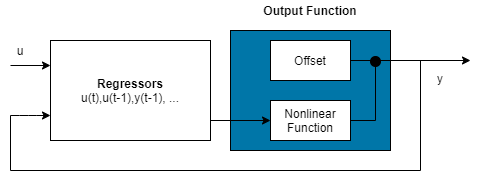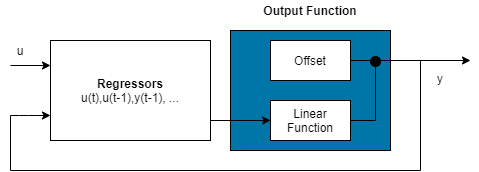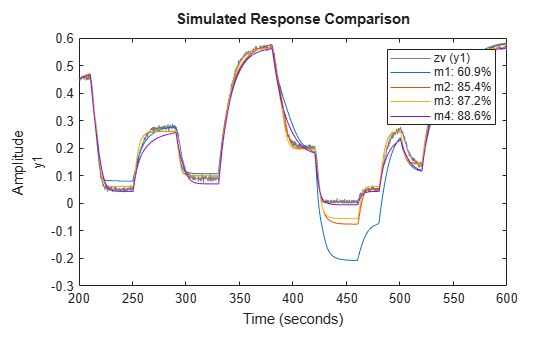Documentation

### This is machine translation

Mouseover text to see original. Click the button below to return to the English version of the page.

## Estimate Nonlinear ARX Models at the Command Line

You can estimate nonlinear ARX models after you perform the following tasks:

### Estimate Model Using `nlarx`

Use `nlarx` to both construct and estimate a nonlinear ARX model. After each estimation, validate the model by comparing it to other models and simulating or predicting the model response.

Basic Estimation

Start with the simplest estimation using `m = nlarx(data,[na nb nk])`. For example:

```load iddata1; % na = nb = 2 and nk = 1 m = nlarx(z1,[2 2 1])```
```m = Nonlinear ARX model with 1 output and 1 input Inputs: u1 Outputs: y1 Standard regressors corresponding to the orders: na = 2, nb = 2, nk = 1 No custom regressor Nonlinear regressors: y1(t-1) y1(t-2) u1(t-1) u1(t-2) Nonlinearity: wavenet with 1 unit Sample time: 0.1 seconds Status: Estimated using NLARX on time domain data "z1". Fit to estimation data: 68.83% (prediction focus) FPE: 1.975, MSE: 1.885 ```

The second input argument `[na nb nk]` specify the model orders and delays. By default, the nonlinearity estimator is the wavelet network (see the `wavenet` reference page), which takes all standard regressors as inputs to its linear and nonlinear functions. `m` is an `idnlarx` object.

For MIMO systems, `nb`, `nf`, and `nk` are ny-by-nu matrices. See the `nlarx` reference page for more information about MIMO estimation.

Specify a different nonlinearity estimator (for example, sigmoid network).

`M = nlarx(z1,[2 2 1],'sigmoid');`

Create an `nlarxOptions` option set and configure the `Focus` property to minimize simulation error.

```opt = nlarxOptions('Focus','simulation'); M = nlarx(z1,[2 2 1],'sigmoid',opt);```

### Configure Model Regressors

Standard Regressors

Change the model order to create a model structure with different model regressors, which are delayed input and output variables that are inputs to the nonlinearity estimator.

Custom Regressors

Explore including custom regressors in the nonlinear ARX model structure. Custom regressors are in addition to the standard model regressors (see Nonlinear ARX Model Orders and Delay).

Use `polyreg` or `customreg` to construct custom regressors in terms of model input-output variables. You can specify custom regressors using the `CustomRegressors` property of the `idnlarx` class or `addreg` to append custom regressors to an existing model.

For example, generate regressors as polynomial functions of inputs and outputs:

```load iddata1 m = nlarx(z1,[2 2 1],'sigmoidnet'); getreg(m) % displays all regressors```
```Regressors: y1(t-1) y1(t-2) u1(t-1) u1(t-2) ```
```% Generate polynomial regressors up to order 2: reg = polyreg(m)```
```4x1 array of Custom Regressors with fields: Function, Arguments, Delays, Vectorized ```

Append polynomial regressors to CustomRegressors.

```m = addreg(m,reg); getreg(m)```
```Regressors: y1(t-1) y1(t-2) u1(t-1) u1(t-2) y1(t-1).^2 y1(t-2).^2 u1(t-1).^2 u1(t-2).^2 ```

`m` now includes polynomial regressors.

You can also specify arbitrary functions of input and output variables. For example:

```load iddata1 m = nlarx(z1,[2 2 1],'sigmoidnet','CustomReg',{'y1(t-1)^2','y1(t-2)*u1(t-3)'}); getreg(m) % displays all regressors```
```Regressors: y1(t-1) y1(t-2) u1(t-1) u1(t-2) y1(t-1)^2 y1(t-2)*u1(t-3) ```

Append polynomial regressors to CustomRegressors.

```m = addreg(m,reg); getreg(m) % polynomial regressors```
```Regressors: y1(t-1) y1(t-2) u1(t-1) u1(t-2) y1(t-1)^2 y1(t-2)*u1(t-3) y1(t-1).^2 y1(t-2).^2 u1(t-1).^2 u1(t-2).^2 ```

Manipulate custom regressors using the `CustomRegressors` property of the `idnlarx` class. For example, to get the function handle of the first custom regressor in the array:

`CReg1 = m.CustomReg(1).Function;`

View the regressor expression.

`m.CustomReg(1).Display`
```ans = 'y1(t-1)^2' ```

You can exclude all standard regressors and use only custom regressors in the model structure by setting `na=nb=nk=0`:

`m = nlarx(z1,[0 0 0],'linear','CustomReg',{'y1(t-1)^2','y1(t-2)*u1(t-3)'});`

In advanced applications, you can specify advanced estimation options for nonlinearity estimators. For example, `wavenet` and `treepartition` classes provide the `Options` property for setting such estimation options.

### Specify Linear and Nonlinear Regressors

By default, all model regressors enter as inputs to both linear and nonlinear function blocks of the nonlinearity estimator. To reduce model complexity and keep the estimation well-conditioned, use a subset of regressors as inputs to the nonlinear function of the nonlinear estimator block. For example, specify a nonlinear ARX model to be linear in past outputs and nonlinear in past inputs.

```m = nlarx(z1,[2 2 1]); % all standard regressors are % inputs to the nonlinear function getreg(m); % lists all standard regressors```
```Regressors: y1(t-1) y1(t-2) u1(t-1) u1(t-2) ```
`m = nlarx(z1,[4 4 1],sigmoidnet,'nlreg',[5 6 7 8]);`

This example uses `getreg` to determine the index of each regressor from the complete list of all model regressors. Only regressor numbers 5 through 8 are inputs to the nonlinear function - `getreg` shows that these regressors are functions of the input variable `u1`. `nlreg` is an abbreviation for the `NonlinearRegressors` property of the `idnlarx` class. Alternatively, include only input regressors in the nonlinear function block using:

`m = nlarx(z1,[4 4 1],sigmoidnet,'nlreg','input');`

When you are not sure which regressors to include as inputs to the nonlinear function block, specify to search during estimation for the optimum regressor combination:

`m = nlarx(z1,[4 4 1],sigmoidnet,'nlreg','search');`

This search typically takes a long time. You can display the search progress using:

```opt = nlarxOptions('Display','on'); m = nlarx(z1,[4 4 1],sigmoidnet,'nlreg','search',opt);```

After estimation, use `m.NonlinearRegressors` to view which regressors were selected by the automatic regressor search.

`m.NonlinearRegressors`
```ans = 1×4 3 5 6 7 ```

### Configure Nonlinearity Estimator

Specify the nonlinearity estimator directly in the estimation command as:

• A character vector of the nonlinearity name, which uses the default nonlinearity configuration.

`m = nlarx(z1, [2 2 1],'sigmoidnet');`
• Nonlinearity object.

`m = nlarx(z1,[2 2 1],wavenet('num',5));`

This estimation uses a nonlinear ARX model with a wavelet nonlinearity that has 5 units. To construct the nonlinearity object before providing it as an input to the nonlinearity estimator:

```w = wavenet('num', 5); m = nlarx(z1,[2 2 1],w); % or w = wavenet; w.NumberOfUnits = 5; m = nlarx(z1,[2 2 1],w);```

For MIMO systems, you can specify a different nonlinearity for each output. For example, to specify `sigmoidnet` for the first output and `wavenet` for the second output:

```load iddata1 z1 load iddata2 z2 data = [z1, z2(1:300)]; M = nlarx(data,[[1 1;1 1] [2 1;1 1] [2 1;1 1]],[sigmoidnet wavenet]);```

If you want the same nonlinearity for all output channels, specify one nonlinearity.

`M = nlarx(data,[[1 1;1 1] [2 1;1 1] [2 1;1 1]],sigmoidnet);`

The following table summarizes values that specify nonlinearity estimators.

NonlinearityValue (Default Nonlinearity Configuration)Class
Wavelet network
(default)
`'wavenet'` or `'wave'``wavenet`
One layer sigmoid network`'sigmoidnet'` or `'sigm'``sigmoidnet`
Tree partition`'treepartition'` or `'tree'``treepartition`
F is linear in x`'linear'` or `[ ]``linear`

Additional available nonlinearities include multilayered neural networks and custom networks that you create.

Specify a multilayered neural network using:

`m = nlarx(data,[na nb nk],NNet)`

where `NNet` is the neural network object you create using the Deep Learning Toolbox™ software. See the `neuralnet` reference page.

Specify a custom network by defining a function called `gaussunit.m`, as described in the `customnet` reference page. Define the custom network object `CNetw` and estimate the model:

```CNetw = customnet(@gaussunit); m = nlarx(data,[na nb nk],CNetw)```

#### Exclude Linear Function in Nonlinearity Estimator

If your model includes `wavenet`, `sigmoidnet`, and `customnet` nonlinearity estimators, you can exclude the linear function using the `LinearTerm` property of the nonlinearity estimator. The nonlinearity estimator becomes F(x)=$g\left(Q\left(x-r\right)\right)$.For example:

```SNL = sigmoidnet('LinearTerm','off'); m = nlarx(z1,[2 2 1],SNL);```

### Note

You cannot exclude the linear function from tree partition and neural network nonlinearity estimators.

#### Exclude Nonlinear Function in Nonlinearity Estimator

Configure the nonlinear ARX structure to include only the linear function in the nonlinearity estimator by setting the nonlinearity to `linear`. In this case, F(x)=${L}^{T}\left(x\right)+d$ is a weighted sum of model regressors plus an offset. Such models provide a bridge between purely linear ARX models and fully flexible nonlinear models.In the simplest case, a model with only standard regressors is linear (affine). For example, this structure:

`m = nlarx(z1,[2 2 1],'linear');`

is similar to the linear ARX model:

`lin_m = arx(z1,[2 2 1]);`

However, the nonlinear ARX model m is more flexible than the linear ARX model `lin_m` because it contains the offset term, d. This offset term provides the additional flexibility of capturing signal offsets, which is not available in linear models.

A popular nonlinear ARX configuration in many applications uses polynomial regressors to model system nonlinearities. In such cases, the system is considered to be a linear combination of products of (delayed) input and output variables. Use the `polyreg` command to easily generate combinations of regressor products and powers.

For example, suppose that you know the output y(t) of a system to be a linear combination of (y(t − 1))2 and y(t − 2)*u(t − 3). To model such a system, use:

`M = nlarx(z1,[0 0 0],'linear','CustomReg',{'y1(t-1)^2','y1(t-2)*u1(t-3)'});`

`M` has no standard regressors and the nonlinearity in the model is described only by the custom regressors.

### Iteratively Refine Model

If your model structure includes nonlinearities that support iterative search (see Specify Estimation Options for Nonlinear ARX Models), you can use `nlarx` to refine model parameters:

```m1 = nlarx(z1,[2 2 1],'sigmoidnet'); m2 = nlarx(z1,m1); % can repeatedly run this command```

You can also use `pem` to refine the original model:

`m2 = pem(z1,m1);`

Check the search termination criterion `m.Report.Termination.WhyStop` . If `WhyStop` indicates that the estimation reached the maximum number of iterations, try repeating the estimation and possibly specifying a larger value for the `nlarxOptions.SearchOptions.MaxIterations` estimation option:

```opt = nlarxOptions; opt.SearchOptions.MaxIterations = 30; m2 = nlarx(z1,m1,opt); % runs 30 more iterations % starting from m1```

When the `m.Report.Termination.WhyStop` value is `Near (local) minimum, (norm( g) < tol` or `No improvement along the search direction with line search` , validate your model to see if this model adequately fits the data. If not, the solution might be stuck in a local minimum of the cost-function surface. Try adjusting the SearchOptions`.Tolerance` value or the `SearchMethod` option in the nlarxOptions option set, and repeat the estimation.

You can also try perturbing the parameters of the last model using `init` (called randomization) and refining the model using `nlarx`:

```M1 = nlarx(z1, [2 2 1], 'sigm'); % original model M1p = init(M1); % randomly perturbs parameters about nominal values M2 = nlarx(z1, M1p); % estimates parameters of perturbed model```

You can display the progress of the iterative search in the MATLAB Command Window using the `nlarxOptions.Display` estimation option:

```opt = nlarxOptions('Display','on'); M2= nlarx(z1,M1p,opt);```

### Troubleshoot Estimation

If you do not get a satisfactory model after many trials with various model structures and algorithm settings, it is possible that the data is poor. For example, your data might be missing important input or output variables and does not sufficiently cover all the operating points of the system.

Nonlinear black-box system identification usually requires more data than linear model identification to gain enough information about the system.

### Use nlarx to Estimate Nonlinear ARX Models

This example shows how to use `nlarx` to estimate a nonlinear ARX model for measured input/output data.

Prepare the data for estimation.

```load twotankdata z = iddata(y, u, 0.2); ze = z(1:1000); zv = z(1001:3000);```

Estimate several models using different model orders, delays, and nonlinearity settings.

```m1 = nlarx(ze,[2 2 1]); m2 = nlarx(ze,[2 2 3]); m3 = nlarx(ze,[2 2 3],wavenet('num',8)); m4 = nlarx(ze,[2 2 3],wavenet('num',8),... 'nlr', [1 2]);```

An alternative way to perform the estimation is to configure the model structure first, and then to estimate this model.

```m5 = idnlarx([2 2 3],sigmoidnet('num',14),'nlr',[1 2]); m5 = nlarx(ze,m5);```

Compare the resulting models by plotting the model outputs with the measured output.

`compare(zv, m1,m2,m3,m4,m5)`